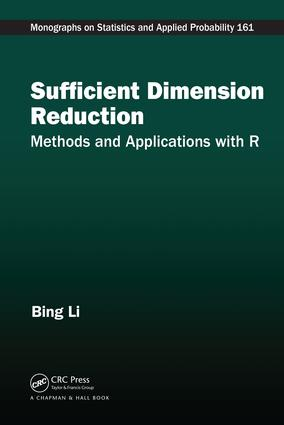Sufficient Dimension Reduction

Methods and Applications with R, 1st Edition

Chapman and Hall/CRC

284 pages | 50 B/W Illus.

Purchasing Options:\$ = USD
Hardback: 9781498704472
pub: 2018-05-01
SAVE ~\$19.99
\$99.95
\$79.96
x
eBook (VitalSource) : 9781315119427
pub: 2018-04-27
from \$49.98

FREE Standard Shipping!

Description

Sufficient dimension reduction is a rapidly developing research field that has wide applications in regression diagnostics, data visualization, machine learning, genomics, image processing, pattern recognition, and medicine, because they are fields that produce large datasets with a large number of variables. Sufficient Dimension Reduction: Methods and Applications with R introduces the basic theories and the main methodologies, provides practical and easy-to-use algorithms and computer codes to implement these methodologies, and surveys the recent advances at the frontiers of this field.

Features

• Provides comprehensive coverage of this emerging research field.
• Synthesizes a wide variety of dimension reduction methods under a few unifying principles such as projection in Hilbert spaces, kernel mapping, and von Mises expansion.
• Reflects most recent advances such as nonlinear sufficient dimension reduction, dimension folding for tensorial data, as well as sufficient dimension reduction for functional data.
• Includes a set of computer codes written in R that are easily implemented by the readers.
• Uses real data sets available online to illustrate the usage and power of the described methods.

Sufficient dimension reduction has undergone momentous development in recent years, partly due to the increased demands for techniques to process high-dimensional data, a hallmark of our age of Big Data. This book will serve as the perfect entry into the field for the beginning researchers or a handy reference for the advanced ones.

The author

Bing Li obtained his Ph.D. from the University of Chicago. He is currently a Professor of Statistics at the Pennsylvania State University. His research interests cover sufficient dimension reduction, statistical graphical models, functional data analysis, machine learning, estimating equations and quasilikelihood, and robust statistics. He is a fellow of the Institute of Mathematical Statistics and the American Statistical Association. He is an Associate Editor for The Annals of Statistics and the Journal of the American Statistical Association.

List of Figures

List of Tables

Foreword

Preface

Author Bios

Contributors

Preliminaries

Empirical Distribution and Sample Moments

Principal Component Analysis

Generalized Eigenvalue Problem

Multivariate Linear Regression

Generalized Linear Model

Exponential family

Generalized Linear Models

Hilbert Space, Linear Manifold, Linear Subspace

Linear Operator and Projection

The Hilbert space Rp(S)

Coordinate Representation

Behavior of Generalized Linear Models under Link Violation

Dimension Reduction Subspaces

Conditional Independence

Sufficient Dimension Reduction Subspace

Behavior of the central subspace under transformations

Fisher Consistency, Unbiasedness, and Exhaustiveness

Sliced Inverse Regression

Sliced Inverse Regression: Population-Level Development

Limitation of SIR

Estimation, Algorithm, and R-codes

Application: the Big Mac index

Parametric and Kernel Inverse Regression

Parametric Inverse Regression

Algorithm, R Codes, and Application

Relation of PIR with SIR

Relation of PIR with Ordinary Least Squares

Kernel Inverse Regression

Sliced Average Variance Estimate

Motivation

Constant Conditional Variance Assumption

Sliced Average Variance Estimate

Algorithm and R-code

Relation with SIR

The Issue of Exhaustiveness

SIR-II

Case Study: The Pen Digit Data

Contour Regression and Directional Regression

Contour Directions and Central Subspace

Contour Regression at the Population Level

Algorithm and R Codes

Exhaustiveness of Contour Regression

Directional Regression

Representation of LDR using moments

Algorithm and R Codes

Exhaustiveness relation with SIR and SAVE

Pen-Digit Case Study Continued

Elliptical Distribution and Transformation of Predictors

Linear Conditional Mean and Elliptical Distribution

Box-Cox Transformation

Application to the Big Mac data

Sufficient Dimension Reduction for Conditional Mean

Central Mean Subspace

Ordinary Least Squares

Principal Hessian Direction

Iterative Hessian Transformation

Asymptotic Sequential Test for Order Determination

Stochastic ordering and von Mises Expansion

von Mises expansion and Influence functions

Influence functions of some useful statistical functionals

Random matrix with Affine invariant eigenvalues

Asymptotic distribution of the sum of small eigenvalues

General form of the sequential tests

Sequential test for SIR

Sequential test for PHD

Sequential test for SAVE

Sequential test for DR

Applications

Other Methods for Order Determination

BIC type criteria for order determination

Order determination by bootstrapped eigenvector variation

Eigenvalue magnitude and eigenvector variation

Consistency of the ladle estimator

Application: identification of wine cultivars

Forward Regressions for Dimension Reduction

Local linear regression and outer product of gradients

Fisher consistency of gradient estimate

Minimum Average Variance Estimate

Refined OPG and MAVE

From central mean subspace to central subspace

dOPG and its refinement

dMAVE and its refinement

Ensemble Estimators

Simulation studies and applications

Summary

Nonlinear Sufficient Dimension Reduction

Reproducing Kernel Hilbert Space

Mean element and covariance operator in RKHS

Coordinate representations

Coordinate of covariance operators

Kernel principal component analysis

Sufficient and central s-field for nonlinear SDR

Complete sub s-field for nonlinear SDR

Converting s-fields to function classes for estimation

Generalized Sliced Inverse Regression

Regression operator

Generalized Sliced Inverse Regression

Exhaustiveness and Completeness

Relative universality

Implementation of GSIR

Precursors and variations of GSIR

Generalized Cross Validation for tuning eX and eY

k-fold Cross Validation for tuning rX ;rY ; eX ; eY

Simulation studies

Applications

Pen Digit data

Face Sculpture data

Generalized Sliced Average Variance Estimator

Generalized Sliced Average Variance Estimation

Relation with GSIR

Implementation of GSAVE

Simulation studies and an application

Relation between linear and nonlinear SDR

Bibliography

Bing Li obtained his Ph.D. from the University of Chicago. He is currently a Professor of Statistics at the Pennsylvania State University. His research interests cover sufficient dimension reduction, statistical graphical models, functional data analysis, machine learning, estimating equations and quasilikelihood, and robust statistics. He is a fellow of the Institute of Mathematical Statistics and the American Statistical Association. He is an Associate Editor for The Annals of Statistics and the Journal of the American Statistical Association.